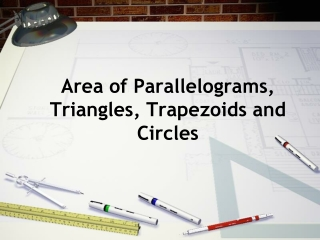DownloadDownload PresentationArea of Parallelograms, Triangles, Trapezoids and Circles

# Area of Parallelograms, Triangles, Trapezoids and Circles

Télécharger la présentation## Area of Parallelograms, Triangles, Trapezoids and Circles

- - - - - - - - - - - - - - - - - - - - - - - - - - - E N D - - - - - - - - - - - - - - - - - - - - - - - - - - -
##### Presentation Transcript

1. Area of Parallelograms, Triangles, Trapezoids and Circles

2. Rectangles and squares are also parallelograms. • The area of a square with side s is A = s2.

3. Example: Find the area of parallelogram in which h = 15 inches and b = 25 inches. A = 375 in2

4. Example: Find the base of the parallelogram in which h = 56 yd and A = 28 yd2. b = 0.5 yd

5. Example: Finding Measurements of Trapezoids Find the area of a trapezoid in which b1 = 8 in., b2 = 5 in., and h = 6.2 in. A = 40.3 in2

6. Example: Finding Measurements of Trapezoids Find b2 of the trapezoid, in which A = 231 mm2. b2 = 19 mm

7. Example: Find the area of the triangle. A = 96 m2

8. Cirmumference C = πd or C=2πr

9. Example: A drum kit contains three drums with diameters of 10 in., 12 in., and 14 in. Find the circumference of each drum. 10 in. diameter 12 in. diameter 14 in. diameter C = d C = d C = d C = (10) C = (12) C = (14) C = 31.4 in. C = 37.7 in. C = 44.0 in.

10. Area of a Circle You can use the circumference of a circle to find its area. Divide the circle and rearrange the pieces to make a shape that resembles a parallelogram. The base of the parallelogram is about half the circumference, or r, and the height is close to the radius r. So A r · r = r2. The more pieces you divide the circle into, the more accurate the estimate will be.

11. A = πr2 Area of a Circle: • Find the area of a circle with a radius of 10 inches. • Find the area of a circle with a diameter of 15 inches. A = 314 in2 A = 176.7 in2